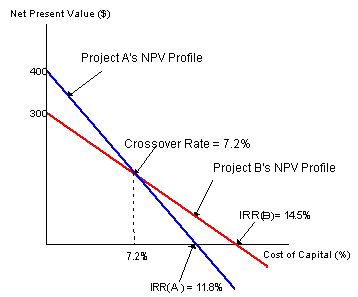### Why should I choose AnalystNotes?

AnalystNotes specializes in helping candidates pass. Period.

##### Subject 4. NPV Profiles
A NPV profile is a graph showing the relationship between a project's NPV and the firm's cost of capital. The point where a project's net present value profile crosses the horizontal axis indicates a project's internal rate of return.Some observations:

• The IRR is the discount rate that sets the NPV to 0.
• The NPV profile declines as the discount rate increases.
• Project A has a higher NPV at low discount rates, while Project B has a higher NPV at high discount rates. The NPV profiles of Project A and B join at the crossover rate, at which the projects' NPVs are equal.
• The slope of Project A's NPV profile is steeper. This indicates that Project A's NPV is more sensitive to changes in the discount rates.

Learning Outcome Statements

e. explain the NPV profile, compare the NPV and IRR methods when evaluating independent and mutually exclusive projects, and describe the problems associated with each of the evaluation methods;

CFA® Level I Curriculum, 2020, Volume 4, Reading 32

User Comment
poomie83 So when the npv crosses beyond the irr horizontal line does that mean npv is less than zero?
sagrr yes, if you choose discount rate above (horizontally) where NPV crosses the horizontal axis, NPV will be less than zero. In this case, for example, for A, a discount rate above 11.8% would result in a negative NPV
bear1234 I was under the impression that NPV can not be less than zero
jonan203 bear1234 - NPV can be any positive or negative value, in practice a project which yields a NPV that is negative would not be selected
darnoor871 This was much easier to understand than the book. Straight forward and to the point.